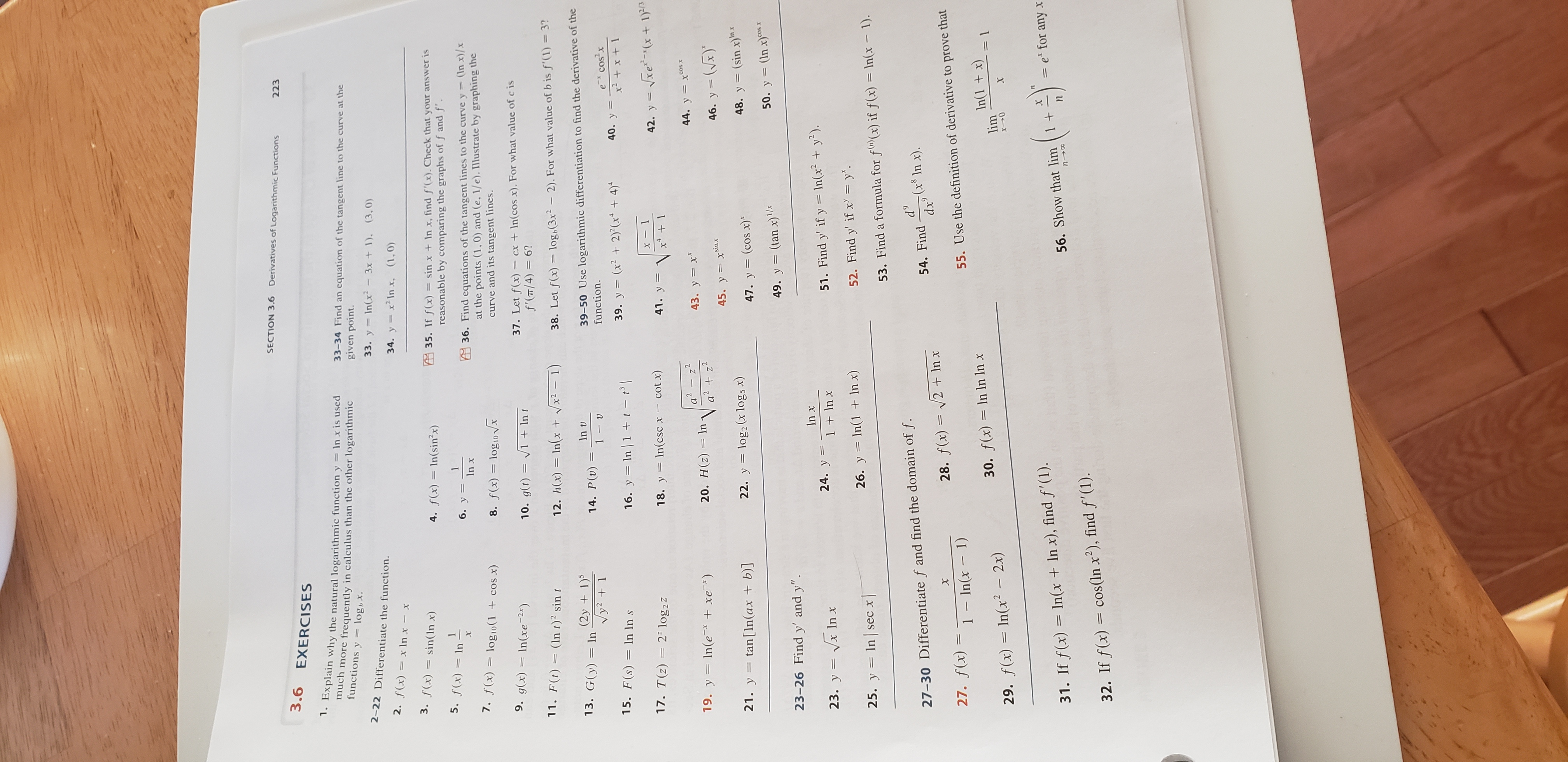# SECTION 3.6Derivatives of Logarithmic Functions2233.6 EXERCISES1. Explain why the natural logarithmic functiony In x is usedmuch more frequently in calculus than the other logarithmicfunctions y33-34 Find an equation of the tangent line to the curve at thegiven point.log,x.33. y In(x 3x +1), (3,0)2-22 Differentiate the function.34. y x2 In x, (1,0)2. f(x)=x In x- x3. f(x)= sin( In x)A35. If f(x) = sin x + In x, find f'(x). Check that your answer isreasonable by comparing the graphs of f andf'.4. f(x)In(sinx)5. f(x)= In16. уX36. Find equations of the tangent lines to the curve y = (In x)/xIn xat the points (1,0) and (e, 1/e). Illustrate by graphing thecurve and its tangent lines.7. f(x)log 10(1 cos x)8. f(x) log10Vx9. g(x)In(xe 2x)37. Let f(x)=f'(T/4) 6?=cx +In(cos x). For what value of c is10. g(t) 1 +Int11. F(t)=(In t) sin t12. h(x) In(x +x21)3?38. Let f(x)= log,(3x2 - 2). For what value of b is f'(1)(2y1)Vy2 139-50 Use logarithmic differentiation to find the derivative of thefunction.13. G(y) InIn v14. P(v)1-e cos x40. у 339. y (x22)(x4)15. F(s) In ln s16. y In 1 + t - t'|х17. T(z)42. y xe(x + 1)22 log2zcot x)18. y In(csc x -41. yx4 1a2 z220. H(z)=In z244. y x43. y x19. y ln(e* + xe_*)46. y (x)45. y xsinxlog2 (x logs x)(sin x)n21. y tan[In(ax + b)]22. y48. y47. y (cos x)*50. y (In x)os49. y (tan x)1/x23-26 Find y' and y"In x24. yV In x51. Find y' if y ln(x2 + y2).23. у 31 + In x52. Find y' if x = y.26. y In(1 + In x)25. y In sec x|53. Find a formula for f((x) if f(x) = In(x - 1).d9(x8 In x)dx27-30 Differentiate f and find the domain of f.54. Find28. f(x) 2 + In xX27. f(x)1 - In(x 1)55. Use the definition of derivative to prove thatIn(1+ x)lim30. f(x) In In In x29. f(x) In(x2 2x)X(0-)-.= ex for any X31. If f(x) In(x + In x), find f'(1).56. Show that lim 1cos (In x2), find f'(1).32. If f(x)

Question

I need help with question 29 in Section 3.6, page 223, of the James Stewart Calculus Eighth Edition textbook.help_outlineImage TranscriptioncloseSECTION 3.6 Derivatives of Logarithmic Functions 223 3.6 EXERCISES 1. Explain why the natural logarithmic functiony In x is used much more frequently in calculus than the other logarithmic functions y 33-34 Find an equation of the tangent line to the curve at the given point. log,x. 33. y In(x 3x +1), (3,0) 2-22 Differentiate the function. 34. y x2 In x, (1,0) 2. f(x)=x In x- x 3. f(x)= sin( In x) A35. If f(x) = sin x + In x, find f'(x). Check that your answer is reasonable by comparing the graphs of f andf'. 4. f(x)In(sinx) 5. f(x)= In 1 6. у X 36. Find equations of the tangent lines to the curve y = (In x)/x In x at the points (1,0) and (e, 1/e). Illustrate by graphing the curve and its tangent lines. 7. f(x) log 10(1 cos x) 8. f(x) log10Vx 9. g(x)In(xe 2x) 37. Let f(x)= f'(T/4) 6? =cx +In(cos x). For what value of c is 10. g(t) 1 +Int 11. F(t)=(In t) sin t 12. h(x) In(x +x21) 3? 38. Let f(x)= log,(3x2 - 2). For what value of b is f'(1) (2y1) Vy2 1 39-50 Use logarithmic differentiation to find the derivative of the function. 13. G(y) In In v 14. P(v) 1- e cos x 40. у 3 39. y (x22)(x4) 15. F(s) In ln s 16. y In 1 + t - t'| х 17. T(z) 42. y xe(x + 1) 22 log2z cot x) 18. y In(csc x - 41. y x4 1 a2 z2 20. H(z)=In z2 44. y x 43. y x 19. y ln(e* + xe_*) 46. y (x) 45. y xsinx log2 (x logs x) (sin x)n 21. y tan[In(ax + b)] 22. y 48. y 47. y (cos x)* 50. y (In x)os 49. y (tan x)1/x 23-26 Find y' and y" In x 24. y V In x 51. Find y' if y ln(x2 + y2). 23. у 3 1 + In x 52. Find y' if x = y. 26. y In(1 + In x) 25. y In sec x| 53. Find a formula for f((x) if f(x) = In(x - 1). d9 (x8 In x) dx 27-30 Differentiate f and find the domain of f. 54. Find 28. f(x) 2 + In x X 27. f(x) 1 - In(x 1) 55. Use the definition of derivative to prove that In(1+ x) lim 30. f(x) In In In x 29. f(x) In(x2 2x) X (0-)-. = ex for any X 31. If f(x) In(x + In x), find f'(1). 56. Show that lim 1 cos (In x2), find f'(1). 32. If f(x) fullscreen
check_circleExpert Solution
Step 1
• Recall d[ln(x)] / dx = 1/x
• Recall the chain rule of differentiation
Step 2

Please see the white board. The expression for f'(x) is the derivative.

Step 3

ln(x) is defined for x>0. Log of a negative number is not defined.

Hence, ln(x2 – 2x) is ...

### Want to see the full answer?

See Solution

#### Want to see this answer and more?

Solutions are written by subject experts who are available 24/7. Questions are typically answered within 1 hour*

See Solution
*Response times may vary by subject and question
Tagged in

### Derivative